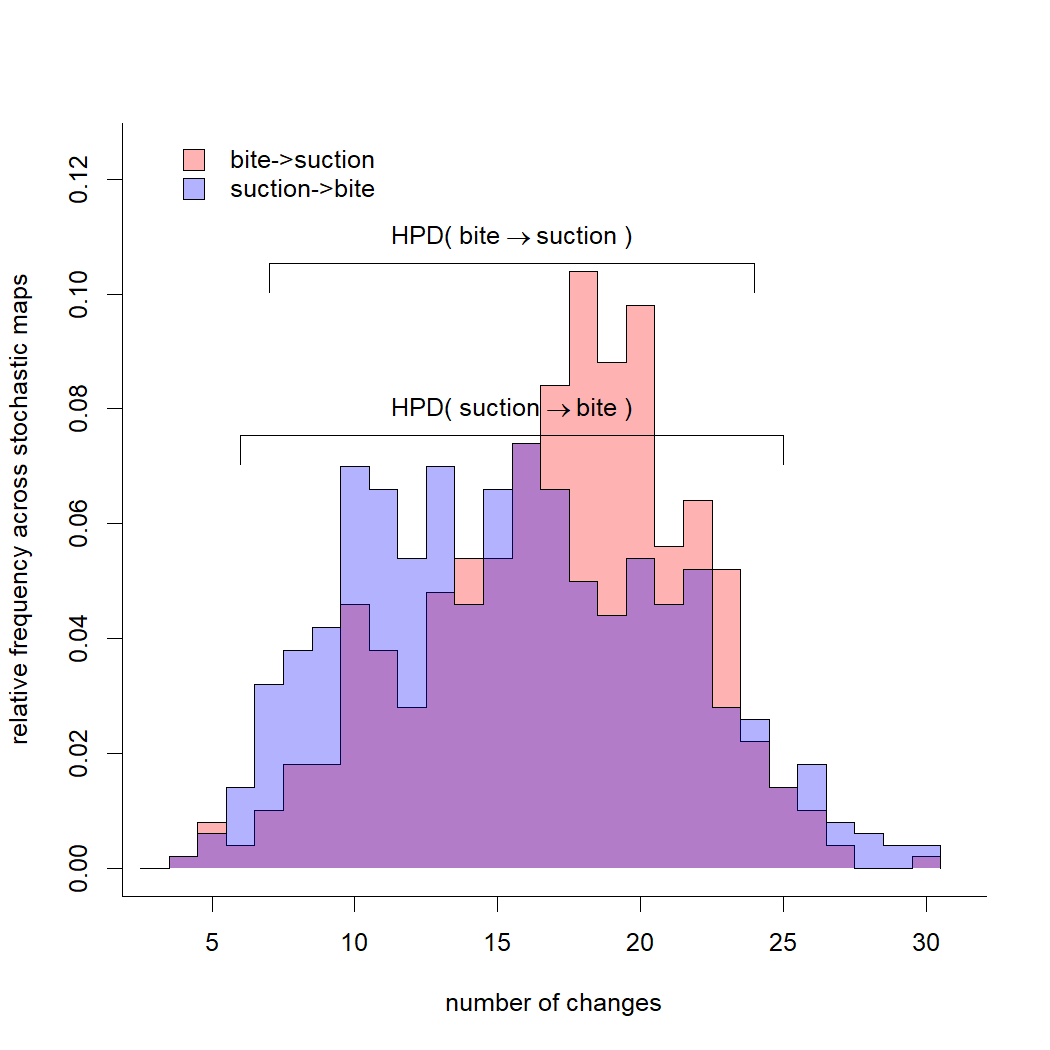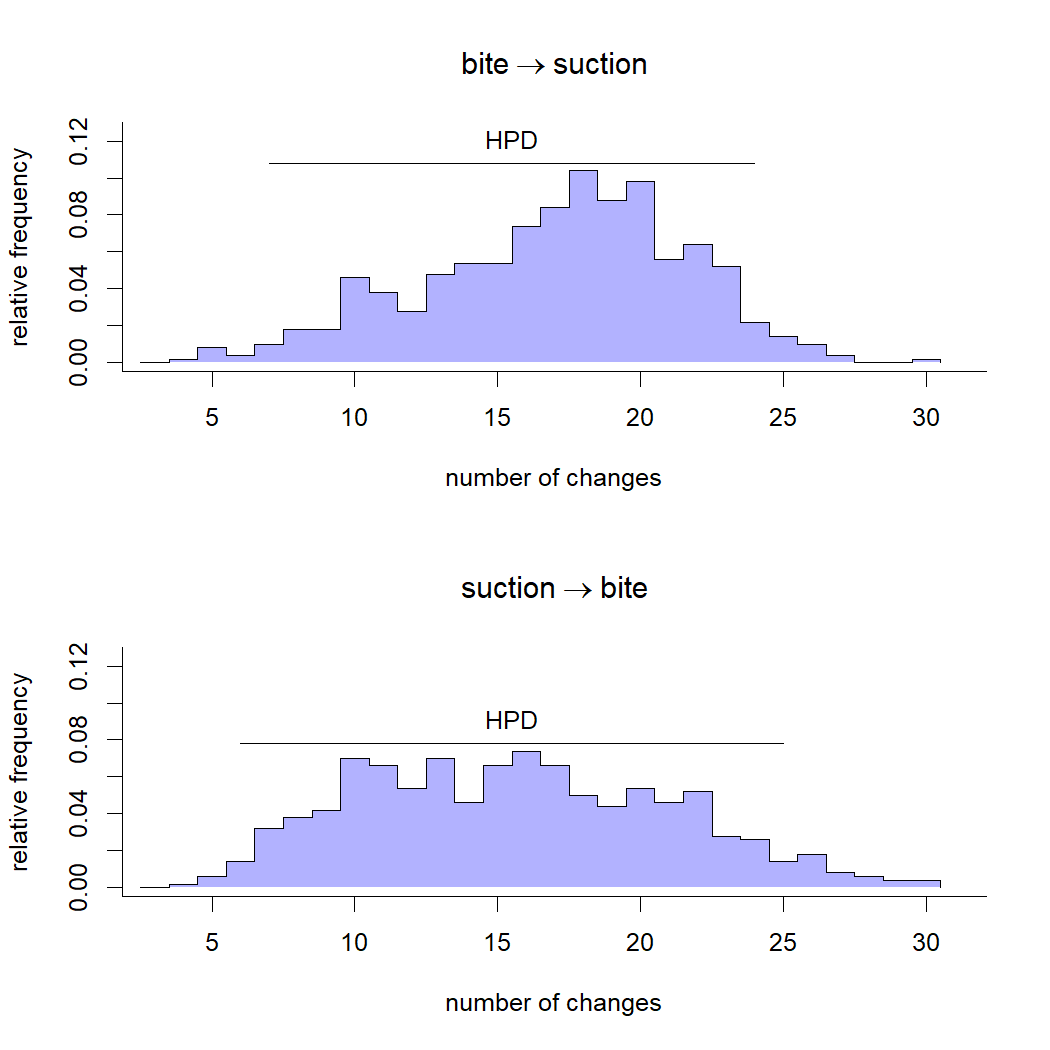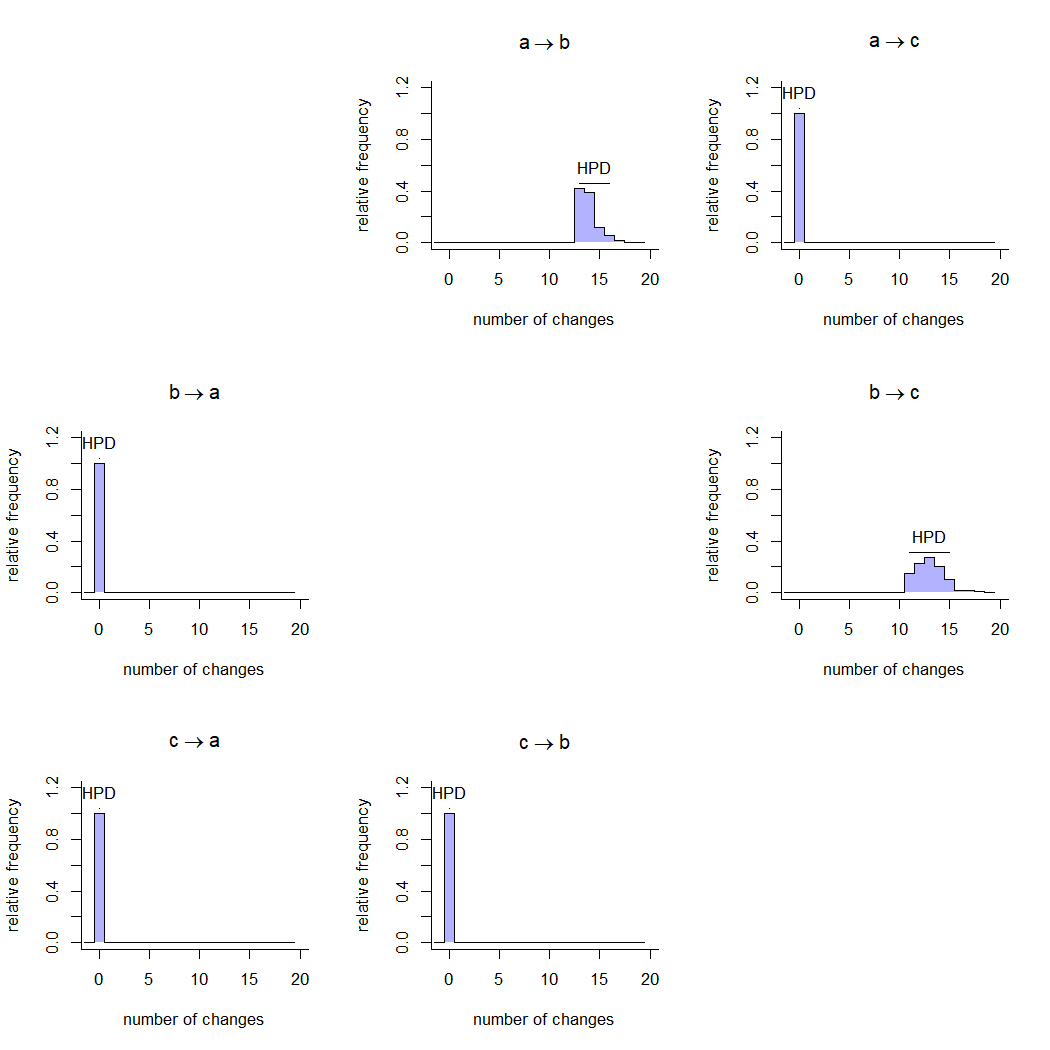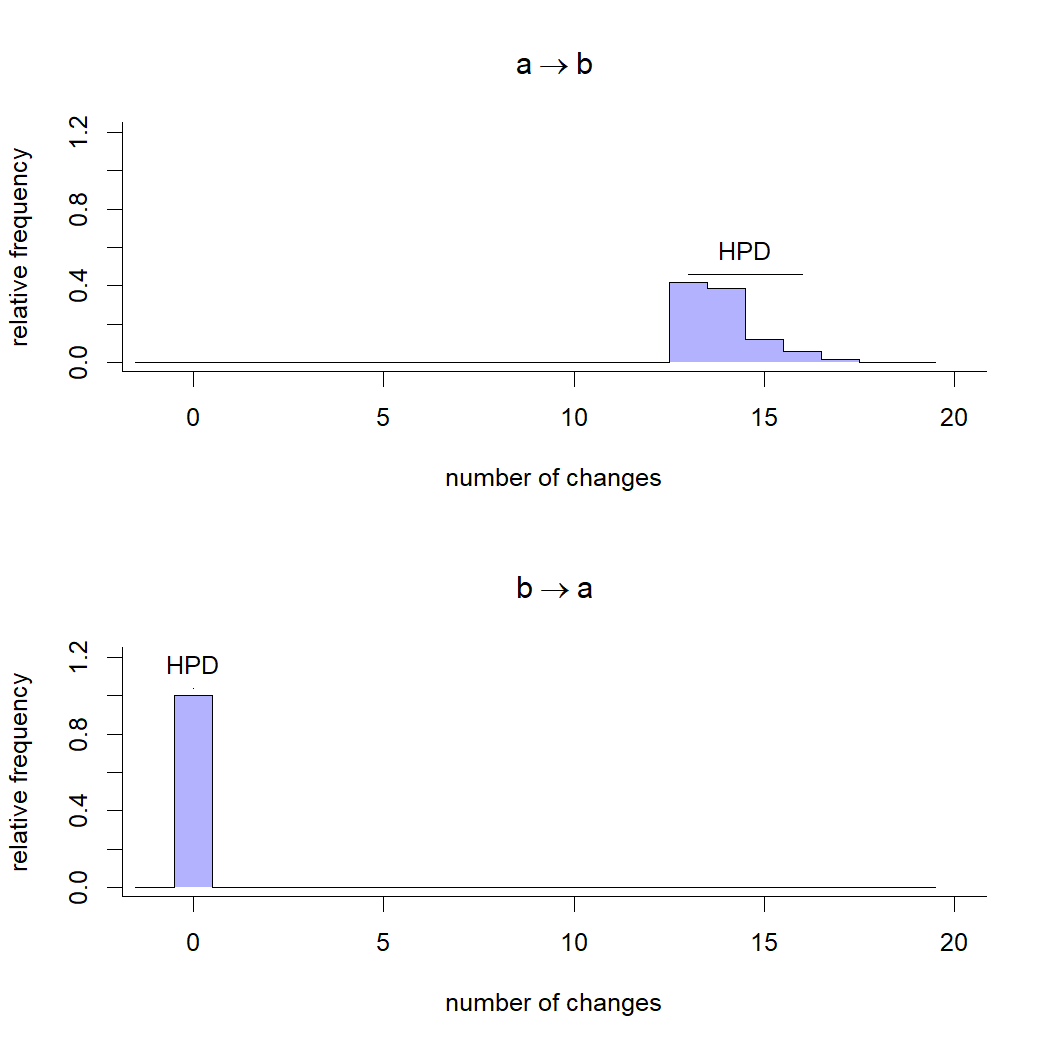## Sunday, May 15, 2022

### Update to plotting method for `"multiSimmap"` density

To better serve a web app that's being developed in my lab, I just added a new feature to the `plot` method associated with the object produced by a call of the function `density` on the `"multiSimmap"` object class.

By default, `density.multiSimmap` computes an object of class `"changesMap"` which can then be graphed to show a posterior distribution of character state changes of different types from the stochastic mapping.

Here's a quick example of what I mean.

First, I'll generate a set of stochastic maps for a discrete character.

``````## load phytools
library(phytools)
## version should be >=1.0.6
packageVersion("phytools")
``````
``````##  '1.0.6'
``````
``````## read tree from file
"http://www.phytools.org/Rbook/8/elopomorph.tre")
eel.tree
``````
``````##
## Phylogenetic tree with 61 tips and 60 internal nodes.
##
## Tip labels:
##   Moringua_edwardsi, Kaupichthys_nuchalis, Gorgasia_taiwanensis, Heteroconger_hassi, Venefica_proboscidea, Anguilla_rostrata, ...
##
## Rooted; includes branch lengths.
``````
``````## read data from file
"http://www.phytools.org/Rbook/8/elopomorph.csv",
stringsAsFactors=TRUE,row.names=1)
``````
``````##                   feed_mode Max_TL_cm
## Albula_vulpes       suction       104
## Anguilla_anguilla   suction        50
## Anguilla_bicolor    suction       120
## Anguilla_japonica   suction       150
## Anguilla_rostrata   suction       152
## Ariosoma_anago      suction        60
``````
``````## extract discrete character
feed_mode<-setNames(eel.data\$feed_mode,rownames(eel.data))
## stochastic mapping
simmap<-make.simmap(eel.tree,feed_mode,nsim=500,
model="ARD",pi="fitzjohn")
``````
``````## make.simmap is sampling character histories conditioned on
## the transition matrix
##
## Q =
##                bite     suction
## bite    -0.01561726  0.01561726
## suction  0.01737975 -0.01737975
## (estimated using likelihood);
## and (mean) root node prior probabilities
## pi =
##      bite   suction
## 0.5148674 0.4851326
``````
``````## Done.
``````
``````simmap
``````
``````## 500 phylogenetic trees with mapped discrete characters
``````

Now I'm going to compute the `density` `"changesMap"` object.

``````density<-density(simmap)
density
``````
``````##
## Distribution of changes from stochastic mapping:
##  bite->suction       suction->bite
##  Min.   :4   Min.   :4
##  Median :18  Median :15
##  Mean   :17.05   Mean   :15.58
##  Max.   :30  Max.   :30
##
## 95% HPD interval(bite->suction): [7, 24]
## 95% HPD interval(suction->bite): [6, 25]
``````

Finally, let's plot it.

``````plot(density)
``````What the update permits is each of the transition types to be graphed separately.

Here's what I mean. I'll actually combine them into a single figure, just to make it look a bit nicer!

``````par(mfrow=c(2,1))
plot(density,transition="bite->suction")
plot(density,transition="suction->bite")
``````To see what happens for a character with more than two states, I'm going to simulate a three level character the same `eel.tree` `"phylo"` object that we've been using.

``````Q<-matrix(c(
-1,1,1,
0,-1,1,
0,0,0),3,3,byrow=TRUE,
dimnames=list(letters[1:3],letters[1:3]))/100
Q
``````
``````##       a     b    c
## a -0.01  0.01 0.01
## b  0.00 -0.01 0.01
## c  0.00  0.00 0.00
``````
``````x<-sim.Mk(eel.tree,Q,anc="a")
simmap<-make.simmap(eel.tree,x,model="ARD",nsim=200,
pi="fitzjohn")
``````
``````## make.simmap is sampling character histories conditioned on
## the transition matrix
##
## Q =
##            a          b         c
## a -0.0110744  0.0110744 0.0000000
## b  0.0000000 -0.0566945 0.0566945
## c  0.0000000  0.0000000 0.0000000
## (estimated using likelihood);
## and (mean) root node prior probabilities
## pi =
## a b c
## 1 0 0
``````
``````## Done.
``````
``````simmap
``````
``````## 200 phylogenetic trees with mapped discrete characters
``````

This is what `density.changesMap` presently does by default with this kind of data.

``````plot(density(simmap))
``````Let's now apply what we just learned to show only changes between states 'a' and 'b', in both and backward directions.

``````par(mfrow=c(2,1))
plot(density(simmap),transition="a->b")
plot(density(simmap),transition="b->a")
``````All right.

#### 1 comment:

1.Hello, I have a question on your "phytools" package.

I wonder this is the right post to comment, but since this is the latest post, I have decided to ask here.
(If this is not appropriate, I will delete this comment and ask it in the right post, sorry.)

I have successfully made 3D phylogenetic tree with the help of your post "New versions of phytools 3D methods"

The script is

library(phytools)
#install.packages("rgl")
library(rgl)
################################
class(tree) <- "phylo"
Bae2 <- matrix(c(1,2,2,3,3,0.5,4,4,5,5,1,4,6,7,9,2,1,1,7,8),10,2,byrow=TRUE)
rownames(Bae2) <- c("un1","un2","un3","un4","un5","un6","un7","un8","un9","un10")
fancyTree(tree, type="traitgram3d",X=Bae2,control=list(spin=TRUE))
################################

The script made 3D phylogenetic tree, but I wanted to apply colors with each branch by their lineage.

While searching for the posts on your blog, I haven't seen any 3D tree with colors.

Is there any way I can apply colors with type="traitgram3d" option?

Thank you.

Note: due to the very large amount of spam, all comments are now automatically submitted for moderation.